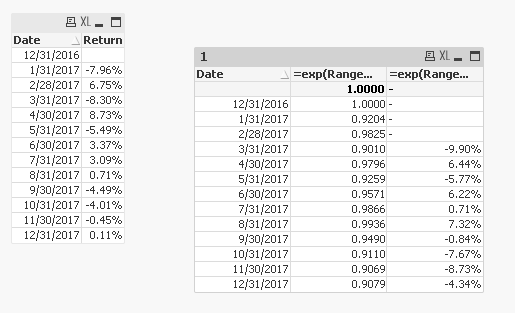# QlikView App Development

Discussion Board for collaboration related to QlikView App Development.

New Contributor III

## Help: Need ideas how to duplicate this excel formula in QlikView?

Can I get some help on how to compute the third column in the attached excel file in QlikView?

• Column F is the Date
• Column G is the percent return say on an investment
• Column H ( Current Index) is the cumulative return  For example H9=H8*(1+G9))

• Column I is where I need help for example cell I15==H15/H12-1 ( this is the 3 month cumulative return for  Sep 30, 2017

Any help will be appreciated. Thanks.

Tags (1)
2 RepliesMVP

## Re: Help: Need ideas how to duplicate this excel formula in QlikView?

For CurentIndex you can try this

=exp(RangeSum(Above(Log(1 + Return), 0, RowNo())))

For 3m Return

=exp(RangeSum(Above(Log(1 + Return), 0, RowNo())))/Above(exp(RangeSum(Above(Log(1 + Return), 0, RowNo()))), 3) - 1MVP

## Re: Help: Need ideas how to duplicate this excel formula in QlikView?

Try this for demonistrator and use same your formula for CurrentIndex

CurrentIndex/Above(exp(RangeSum(Below(-Log(1 + Return), 0, RowNo(TOTAL)))), 3)

Before develop something, think If placed (The Right information | To the right people | At the Right time | In the Right place | With the Right context)